## Data-Structures

 Question 1
Consider the following statements:
I. The smallest element in a max-heap is always at a leaf node.
II. The second largest element in a max-heap is always a child of the root node.
III. A max-heap can be constructed from a binary search tree in Θ(n) time.
IV. A binary search tree can be constructed from a max-heap in Θ(n) time.
Which of the above statements are TRUE?
 A I, II and III B II, III and IV C I, III and IV D I, II and IV
Data-Structures       Binary-Trees       GATE 2019       Video-Explanation
Question 1 Explanation:
i) TRUE: The smallest element in heap is always a leaf node but depends upon the graph, it may be left or right side of the graph.
(ii) TRUE: The second smallest element in a heap is always a child of root node.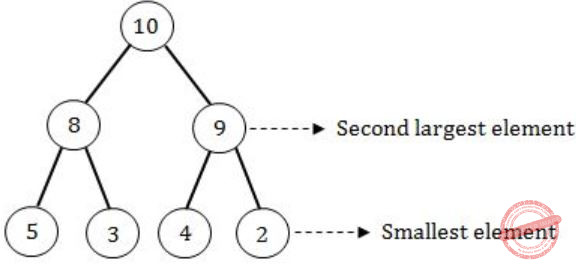(iii) TRUE: Converting from binary search tree to max heap will take O(n) time as well as O(n) space complexity.
(iv) FALSE: We can’t convert max heap to binary search tree in O(n) time.
 Question 2

Let T be a full binary tree with 8 leaves. (A full binary tree has every level full). Suppose two leaves a and b of T are chosen uniformly and independently at random. The expected value of the distance between a and b in T (i.e., the number of edges in the unique path between a and b) is (rounded off to 2 decimal places) _____.

 A 5.54 B 1.34 C 4.25 D 3.82
Data-Structures       Binary-Trees       GATE 2019       Video-Explanation
Question 2 Explanation:
There can be 8 paths between any 2 uniformly & independently chosen leaf nodes.
A node can be chosen twice and the path from that node to itself will be zero.
∴ Path 1 = 0
Similarly,
Path 2 = 2
Path 3 = 4
Path 4 = 4
Path 5 = 6
Path 6 = 6
Path 7 = 6
Path 8 = 6
∴ Expected value = Σ Path length × Probability of selecting path
= 2×1/8 + 4×2/8 + 6×4/8 + 0×1/8
= 1/4 + 1/1 + 3/1 + 0
= 4 + 1/4
= 17/4
= 4.25
 Question 3

The postorder traversal of a binary tree is 8, 9, 6, 7, 4, 5, 2, 3, 1. The inorder traversal of the same tree is 8, 6, 9, 4, 7, 2, 5, 1, 3. The height of a tree is the length of the longest path from the root to any leaf. The height of the binary tree above is _______.

 A 1 B 2 C 3 D 4
Data-Structures       Trees       GATE 2018       Video-Explanation
Question 3 Explanation:
Post – 8 9 6 7 4 5 2 3 1
In – 8 6 9 4 7 2 5 1 3
Post: 8 9 6 7 4 5 2 3 1→(root)
In: 8 6 9 4 7 2 5→(left subtree) 13→(right subtree)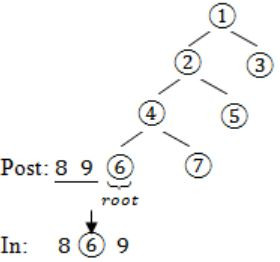Question 4

A queue is implemented using a non-circular singly linked list. The queue has a head pointer and a tail pointer, as shown in the figure. Let n denote the number of nodes in the queue. Let ‘enqueue’ be implemented by inserting a new node at the head, and ‘dequeue’ be implemented by deletion of a node from the tail.Which one of the following is the time complexity of the most time-efficient implementation of ‘enqueue’ and ‘dequeue, respectively, for this data structure?

 A θ(1), θ(1) B θ(1), θ(n) C θ(n), θ(1) D θ(n), θ(n)
Question 4 Explanation:
For insertion of node at the beginning of linked list only need manipulation of pointers so θ(1) time.
But if we have pointer to the tail of the list in order to delete it, we need the address of the 2nd last node which can be obtained by traversing the list which takes O(n) time.
 Question 5
Let G be a simple undirected graph. Let TD be a depth first search tree of G. Let TB be a breadth first search tree of G. Consider the following statements.
(I) No edge of G is a cross edge with respect to TD.(A cross edge in G is between two nodes neither of which is an ancestor of the other in TD.)
(II) For every edge (u,v) of G, if u is at depth i and v is at depth j in TB, then ∣i−j∣ = 1.
Which of the statements above must necessarily be true?
 A I only B II only C Both I and II D Neither I nor II
Data-Structures       Graphs       GATE 2018       Video-Explanation
Question 5 Explanation:
I. Undirected graph do not have cross edges in DFS. But can have cross edges in directed graph. Hence True.
II. Just draw a triangle ABC. Source is A. Vertex B and C are at same level at distance 1.
There is an edge between B and C too. So here |i – j| = |1 – 1| = 0. Hence, False.
 Question 6

The number of possible min-heaps containing each value from {1, 2, 3, 4, 5, 6, 7} exactly once is ___________.

 A 80 B 81 C 82 D 83
Data-Structures       Heap-Tree       GATE 2018       Video-Explanation
Question 6 Explanation:
–> We have 7 distinct integers {1,2,3,4,5,6,7} and sort it
–> After sorting, pick the minimum element and make it the root of the min heap.
–> So, there is only 1 way to make the root of the min heap.
–> Now we are left with 6 elements.
–> Total ways to design a min heap from 6 elements = C(6,3) ∗ 2! ∗ C(3,3) ∗ 2! = 80

Note:
C(6,3)∗2! : Pick up any 3 elements for the left subtree and each left subtree combination can be permuted in 2! ways by interchanging the children and similarly, for right subtree .
 Question 7

Consider the C code fragment given below.

```typedef struct node   {
int data;
node* next;
}   node;

void join(node* m, node* n)   {
node*  p = n;
while(p→next  !=NULL)    {
p = p→next;
}
p→next=m;
}
```

Assuming that m and n point to valid NULL-terminated linked lists, invocation of join will

 A append list m to the end of list n for all inputs. B either cause a null pointer dereference or append list m to the end of list n. C cause a null pointer dereference for all inputs. D append list n to the end of list m for all inputs.
Data-Structures       Linked-List       GATE 2017 [Set-1]       Video-Explanation
Question 7 Explanation:
As specified in the question m & n are valid lists, but there is no specific condition/ statement tells that lists are empty or not.
So have to consider both the cases.
⇾ 1. Lists are not null, invocation of JOIN will append list m to the end of list n.
m = 1, 2, 3
n = 4, 5, 6
After join, it becomes 4, 5, 6, 1, 2, 3, NULL.
⇾ 2. If lists are null, if the list n is empty, and itself NULL, then joining & referencing would obviously create a null pointer issue.
Hence, it may either cause a NULL pointer dereference or appends the list m to the end of list n.
 Question 8

Let T be a tree with 10 vertices. The sum of the degrees of all the vertices in T is _________.

 A 18 B 19 C 20 D 21
Data-Structures       Trees       GATE 2017 [Set-1]       Video-Explanation
Question 8 Explanation:
Sum of degrees of all vertices is double the number of edges.
A tree, with 10 vertices, consists n – 1, i.e. 10 – 1 = 9 edges.
Sum of degrees of all vertices = 2(#edges)
= 2(9)
= 18
 Question 9
A circular queue has been implemented using a singly linked list where each node consists of a value and a single pointer pointing to the next node. We maintain exactly two external pointers FRONT and REAR pointing to the front node and the rear node of the queue, respectively. Which of the following statements is/are CORRECT for such a circular queue, so that insertion and deletion operations can be performed in O(1) time?
I. Next pointer of front node points to the rear node.
II. Next pointer of rear node points to the front node.
 A I only B II only C Both I and II D Neither I nor II
Data-Structures       Circular-Queue-and-Linked-List       GATE 2017 [Set-2]       Video-Explanation
Question 9 Explanation:
1. Next pointer of front node points to the rear node.
2. Next pointer of rear points to the front node.So if we consider circular linked list the next of 44 is 11.
If you place pointer on next of front node (11) then to reach 44 (last node), we have to traverse the entire list.
For delete O(1), for insert O(n).
It is clearly known that next pointer of rear node points to the front node.
Hence, only II is true.
 Question 10

The Breadth First Search (BFS) algorithm has been implemented using the queue data structure. Which one of the following is a possible order of visiting the nodes in the graph below?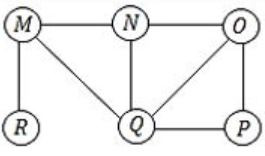A MNOPQR B NQMPOR C QMNROP D POQNMR
Data-Structures       BFS       GATE 2017 [Set-2]       Video-Explanation
Question 10 Explanation:
The possible order of visiting the nodes in Breadth First Search Algorithm, implementing using Queue Data Structure is(Do it by option Elimination)
(a) MNOPQR – MNO is not the proper order R must come in between.
(b) NQMPOR – QMP is not the order O is the child of N.
(C) QMNROP – M is not the child of Q, so QMN is false.
(D) POQNMR – P → OQ → NMR is the correct sequence. Hence Option (D).
 Question 11

The pre-order traversal of a binary search tree is given by 12, 8, 6, 2, 7, 9, 10, 16, 15, 19, 17, 20. Then the post-order traversal of this tree is:

 A 2, 6, 7, 8, 9, 10, 12, 15, 16, 17, 19, 20 B 2, 7, 6, 10, 9, 8, 15, 17, 20, 19, 16, 12 C 7, 2, 6, 8, 9, 10, 20, 17, 19, 15, 16, 12 D 7, 6, 2, 10, 9, 8, 15, 16, 17, 20, 19, 12
Data-Structures       Binary-Trees       GATE 2017 [Set-2]       Video-Explanation
Question 11 Explanation:From these 2 orders, we can say 12 is the root & 8 is the root of left subtree & 16 is the root of right subtree.From 2, 6, 7 we can identify 6 is the root from preorder traversal and from 9, 10 → 9 is root.
From <17, 19, 20>, 19 as root.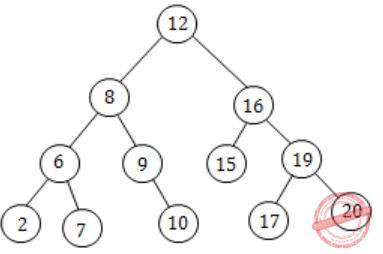Hence, 2,7,6,10,9,8 |,15,17,20,19,16 |12 is the post-order traversal.
 Question 12

A queue is implemented using an array such that ENQUEUE and DEQUEUE operations are performed efﬁciently. Which one of the following statements is CORRECT (n refers to the number of items in the queue)?

 A Both operations can be performed in O(1) time B At most one operation can be performed in O(1) time but the worst case time for the other operation will be Ω(n) C The worst case time complexity for both operations will be Ω(n) D Worst case time complexity for both operations will be Ω(logn)
Data-Structures       Queues       GATE 2016 [Set-1]       Video-Explanation
Question 12 Explanation:
Since it is mentioned in the question that both of the operations are performed efficiently.
Hence even the worst case time complexity will be O(1) by the use of the Circular queue there won’t be any need of shifting in the array.
 Question 13

Consider the following directed graph: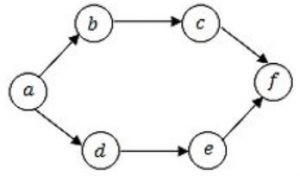The number of different topological orderings of the vertices of the graph is __________.

 A 7 B 9 C 8 D 6
Data-Structures       Topological ordering       GATE 2016 [Set-1]       Video-Explanation
Question 13 Explanation:
Different topological orderings of the vertices of the graph are:It is observed that (a) is the starting vertex & (f) is the final one.
Also observed that c must come after b & e must come after d.
So,Hence, there are 6 different topological orderings can be derived.
 Question 14
Let G be a weighted connected undirected graph with distinct positive edge weights. If every edge weight is increased by the same value, then which of the following statements is/are TRUE?
P: Minimum spanning tree of G does not change
Q: Shortest path between any pair of vertices does not change
 A P only B Q only C Neither P nor Q D Both P and Q
Data-Structures       Graphs       GATE 2016 [Set-1]       Video-Explanation
Question 14 Explanation:
Given undirected weighted graph with distinct positive edges.
Every edge weight is increased by the same value say,P: Minimum Spanning Tree will not change ABC in both the cases.
Q: Shortest path will change because in 1st figure the path from A to C calculated as ABC but in fig.2, it is AC (Direct path).
 Question 15

An operator delete(i) for a binary heap data structure is to be designed to delete the item in the i-th node. Assume that the heap is implemented in an array and i refers to the i-th index of the array. If the heap tree has depth d (number of edges on the path from the root to the farthest leaf), then what is the time complexity to re-ﬁx the heap efﬁciently after the removal of the element?

 A O(1) B O(d) but not O(1) C O(2d) but not O(d) D O(d 2d) but not O(2d)
Data-Structures       Binary-Heap       GATE 2016 [Set-1]       Video-Explanation
Question 15 Explanation:
→ If heap has n elements generally it takes O(n) to delete any arbitrary element.
→ Because we first need to find that element, O(n) time
Delete that element O(height) [deleting involves swapping particular element to rightmost leaf and then do heapify on that node].
**but here, we don’t need to find that element, because in delete(i), i is index of that element.
Note: Delete time = O(height) = O(d)
 Question 16

Consider the weighted undirected graph with 4 vertices, where the weight of edge {i,j} is given by the entry Wij  in the matrix W.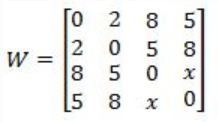The largest possible integer value of x, for which at least one shortest path between some pair of vertices will contain the edge with weight x is _________.

 A 12 B 13 C 14 D 15
Data-Structures       Graphs       GATE 2016 [Set-1]       Video-Explanation
Question 16 Explanation:
Let vertices be A, B, C and D.
x directly connects C to D.
The shortest path (excluding x) from C to D is of weight 12 (C-B-A-D).
 Question 17

Let Q denote a queue containing sixteen numbers and S be an empty stack. Head(Q) returns the element at the head of the queue Q without removing it from Q. Similarly Top(S) returns the element at the top of S without removing it from S. Consider the algorithm given below.The maximum possible number of iterations of the while loop in the algorithm is _________.

 A 256 B 257 C 258 D 259
Data-Structures       Queues-and-Stacks       GATE 2016 [Set-1]       Video-Explanation
Question 17 Explanation:
The maximum possible number of iterations of the while loop in this algorithm is:
Try to solve it for 3 numbers [1. 2, 3].
Step 1: Initially Queue contains 3 elements so after 5 while loop iterations queue contains 3, 2 and stack contains 1.
Step 2: Now after 3 more while loop iterations, Queue contains 3 and stack contains 1, 2 (TOS = 2).
Step 3: After 1 more while loop iteration, push 3 onto the stack so queue is empty and stack contains 1, 2, 3 {top = 3}.
So, total number of iterations will be 5 + 3 + 1 = 9
i.e., for 3 it is 9 iterations (3*3)
for 4 it is 16 iterations (4*4)
Given: 16 numbers, so 16 * 16 = 256
 Question 18

Breadth First Search (BFS) is started on a binary tree beginning from the root vertex. There is a vertex t at a distance four from the root. If t is the n-th vertex in this BFS traversal, then the maximum possible value of n is _________.

 A 31 B 32 C 33 D 34
Data-Structures       BFS       GATE 2016 [Set-2]       Video-Explanation
Question 18 Explanation:
Given is a vertex t at a distance of 4 from the root.For distance 1, max possible value is (3).
Similarly, for distance 2, max value is (7).
So, maximum number of nodes = 2(h+1) – 1
For distance 4, 2(4+1) – 1 ⇒ 25 – 1 ⇒ 32 – 1 = 31
31 is the last node.So for distance 4, the maximum possible node will be 31 & minimum will be 16.
 Question 19

N items are stored in a sorted doubly linked list. For a delete operation, a pointer is provided to the record to be deleted. For a decrease-key operation, a pointer is provided to the record on which the operation is to be performed.

An algorithm performs the following operations on the list in this order: Θ(Ndelete,O(logN) insert, O(logN) ﬁnd, and Θ(N) decrease-key. What is the time complexity of all these operations put together?

 A O(log2 N) B O(N) C O(N2) D Θ(N2 logN)
Data-Structures       Linked-List       GATE 2016 [Set-2]       Video-Explanation
Question 19 Explanation:
→ Delete needs O(1) time
→ Insert takes O(N) time
→ Find takes O(N) time
→ Decrease by takes O(N) time
Now number of each operation performed is given, so finally total complexity,
→ Delete = O(1) × O(N) = O(N)
→ Find = O(N) × O(log N) = O(N log N)
→ Insert = O(N) × O(log N) = O(N log N)
→ Decrease key = O(N) × O(N) = O(N2)
So, overall time complexity is, O(N2).
 Question 20

A complete binary min-heap is made by including each integer in [1, 1023] exactly once. The depth of a node in the heap is the length of the path from the root of the heap to that node. Thus, the root is at depth 0. The maximum depth at which integer 9 can appear is _________.

 A 8 B 9 C 10 D 11
Data-Structures       Heap-Tree       GATE 2016 [Set-2]       Video-Explanation
Question 20 Explanation:This is not possible because it violates a property of complete binary tree.
We have total [0, 1023] elements. It means that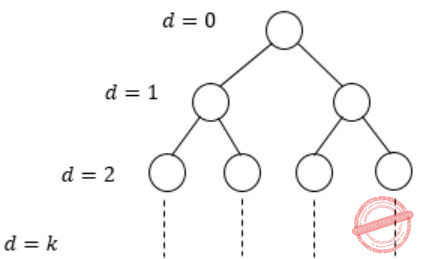∴ 20 + 21 + 22 + ⋯ + 2k = 1024
Add if 1(2(k+1)-1)/(2-1) [using formula for sum of k terms k+1 in G.P]
= 2(k+1) – 1 = 1024 – 1 = 1023
∴ The level ‘9’ at the depth of 8.
Actually we have 1023 elements, we can achieve a complete binary min heap of depth 9, which would cover all 1023 elements, but the max depth of node 9 can be only be 8.
 Question 21

Consider the following New-order strategy for traversing a binary tree:

• Visit the root;
• Visit the right subtree using New-order;
• Visit the left subtree using New-order;

The New-order traversal of the expression tree corresponding to the reverse polish expression 3 4 * 5 – 2 ˆ 6 7 * 1 + – is given by:

 A + – 1 6 7 * 2 ˆ 5 – 3 4 * B – + 1 * 6 7 ˆ 2 – 5 * 3 4 C – + 1 * 7 6 ˆ 2 – 5 * 4 3 D 1 7 6 * + 2 5 4 3 * – ˆ –
Data-Structures       Binary-Trees       GATE 2016 [Set-2]       Video-Explanation
Question 21 Explanation:
New Order strategy: Root, Right, Left.
Given Reverse Polish Notation as:
3 4 * 5 – 2 ^ 6 7 * 1 + –
We know Reverse Polish Notation takes Left, Right, Root.So the expression tree looks likeFrom the tree, we can write the New Order traversal as: Root, Right, Left.
– + 1 * 7 6 ^ 2 – 5 * 4 3
 Question 22

The number of ways in which the numbers 1, 2, 3, 4, 5, 6, 7 can be inserted in an empty binary search tree, such that the resulting tree has height 6, is _________.

Note: The height of a tree with a single node is 0.
 A 64 B 65 C 66 D 67
Data-Structures       Trees       GATE 2016 [Set-2]       Video-Explanation
Question 22 Explanation:
To get the tree of height 6, every level should contain only 1 node.
So to get such tree at each level we should have either maximum or minimum element from remaining numbers till that level. So no. of binary search tree possible is,
1st level – 2 (maximum or minimum)

2nd level – 2

3rd level – 2

4th level – 2

5th level – 2

6th level – 2

7th level – 2
= 2 × 2 × 2 × 2 × 2 × 2 × 1
= 26
= 64
 Question 23

In an adjacency list representation of an undirected simple graph G = (V,E), each edge (u,v) has two adjacency list entries: [v] in the adjacency list of u, and [u] in the adjacency list of v. These are called twins of each other. A twin pointer is a pointer from an adjacency list entry to its twin. If |E| = m and |V| = n, and the memory size is not a constraint, what is the time complexity of the most efﬁcient algorithm to set the twin pointer in each entry in each adjacency list?

 A Θ(n2) B Θ(n+m) C Θ(m2) D Θ(n4)
Data-Structures       Graphs       GATE 2016 [Set-2]       Video-Explanation
Question 23 Explanation:
Applying BFS on Undirected graph give you twin pointer.
Visit every vertex level-wise for every vertex fill adjacent vertex in the adjacency list. BFS take O(m+n) time.
Note:
Twin Pointers can be setup by keeping track of parent node in BFS or DFS of graph.
 Question 24

The height of a tree is the length of the longest root-to-leaf path in it. The maximum and minimum number of nodes in a binary tree of height 5 are

 A 63 and 6, respectively B 64 and 5, respectively C 32 and 6, respectively D 31 and 5, respectively
Data-Structures       Trees       GATE 2015 [Set-1]
Question 24 Explanation:
Maximum number of nodes in a binary tree of height h is,
2h+1 – 1 = 25+1 – 1 = 63
Minimum number of nodes in a binary tree of height h is
h + 1 = 5 + 1 = 6
 Question 25

Consider a max heap, represented by the array: 40, 30, 20, 10, 15, 16, 17, 8, 4.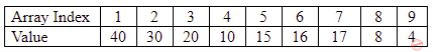Now consider that a value 35 is inserted into this heap. After insertion, the new heap is

 A 40, 30, 20, 10, 15, 16, 17, 8, 4, 35 B 40, 35, 20, 10, 30, 16, 17, 8, 4, 15 C 40, 30, 20, 10, 35, 16, 17, 8, 4, 15 D 40, 35, 20, 10, 15, 16, 17, 8, 4, 30
Data-Structures       Heap       GATE 2015 [Set-1]
Question 25 Explanation:
Given max. heap isHeapification: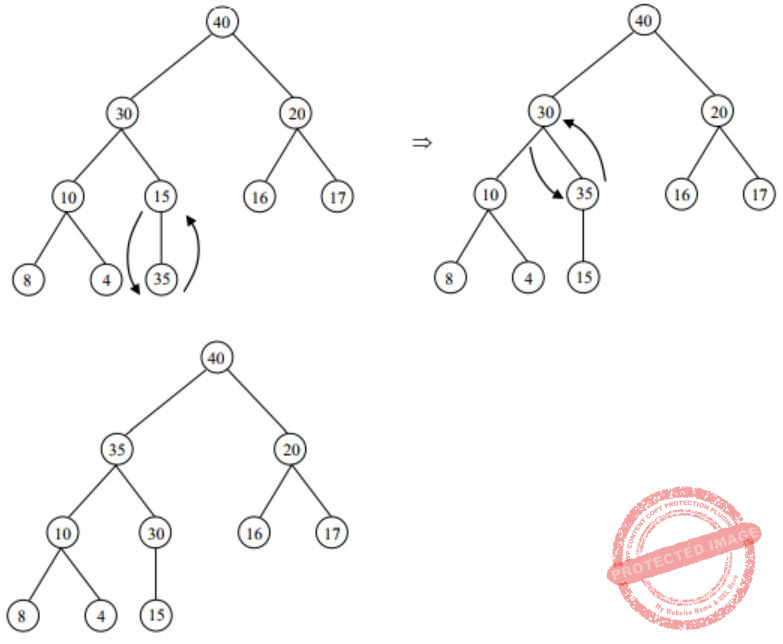Array representation of above max-heap is (BFS)
40, 35, 20, 10, 30, 16, 17, 8, 4, 15
 Question 26

A binary tree T has 20 leaves. The number of nodes in T having two children is _________.

 A 19 B 20 C 21 D 22
Data-Structures       Binary-Trees       GATE 2015 [Set-2]
Question 26 Explanation:
Let the number of vertices of a binary tree with “p” leaves be n then the tree has
(i) p vertices (i.e., leaves) of degree 1
(ii) one vertex (i.e., root of T) of degree 2
(iii) ‘n – p – 1’ (i.e., interval) vertices of degree 3
(iv) n – 1 edges
∴ By Handshaking theorem,
p × 1 + 1 × 2 + (n – p – 1) × 3 = 2(n – 1)
⇒n = 2p – 1
= 39 as p = 20
∴ n – p = 19 vertices have exactly two children
 Question 27

Consider a complete binary tree where the left and the right subtrees of the root are max-heaps. The lower bound for the number of operations to convert the tree to a heap is

 A Ω(logn) B Ω(n) C Ω(nlog n) D Ω(n2)
Data-Structures       Heap-Tree       GATE 2015 [Set-2]
Question 27 Explanation:
Since left and right subtree is already a heap. So we can apply Heapify (node) which take log n time.
 Question 28

Which one of the following hash functions on integers will distribute keys most uniformly over 10 buckets numbered 0 to 9 for i ranging from 0 to 2020?

 A h(i) = i2 mod 10 B h(i) = i3 mod 10 C h(i) = (11 *i2) mod 10 D h(i) = (12 * i) mod 10
Data-Structures       Hashing       GATE 2015 [Set-2]
Question 28 Explanation:
If we take first 10 elements, number of collisions taken by the hash function given by option (B) is less when compared to others.
 Question 29

Assume that the bandwidth for a TCP connection is 1048560 bits/sec. Let α be the value of RTT in milliseconds (rounded off to the nearest integer) after which the TCP window scale option is needed. Let β be the maximum possible window size the window scale option. Then the values of α and β are

 A 63 milliseconds, 65535×214 B 63 milliseconds, 65535×216 C 500 milliseconds, 65535×214 D 500 milliseconds, 65535×216
Data-Structures       TCP       GATE 2015 [Set-2]
Question 29 Explanation:
TCP header sequence number field consist 16 bits. The maximum number of sequence numbers possible = 216 = 65,535.
The wrap around time for given link = 1048560 * α. The TCP window scale option is an option to increase the receive window size. TCP allows scaling of windows when wrap around time > 65,535.
==> 1048560 * α > 65,535*8 bits
==> α = 0.5 sec = 500 mss
Scaling is done by specifying a one byte shift count in the header options field. The true receiver window size is left shifted by the value in shift count. A maximum value of 14 may be used for the shift count value. Therefore maximum window size with scaling option is 65535 × 214.
 Question 30

A Young tableau is a 2D array of integers increasing from left to right and from top to bottom. Any unfilled entries are marked with ∞, and hence there cannot be any entry to the right of, or below a ∞. The following Young tableau consists of unique entries.

```1     2     5      14
3     4     6      23
10    12    18     25
31    ∞     ∞       ∞ ```

When an element is removed from a Young tableau, other elements should be moved into its place so that the resulting table is still a Young tableau (unfilled entries may be filled in with a ∞). The minimum number of entries (other than 1) to be shifted, to remove 1 from the given Young tableau is ____________.

 A 4 B 5 C 6 D 7
Data-Structures       Arrays       GATE 2015 [Set-2]
Question 30 Explanation:Question 31

Given a hash table T with 25 slots that stores 2000 elements, the load factor α for T is ___________.

 A 80 B 70 C 60 D 50
Data-Structures       Hashing       GATE 2015 [Set-3]
Question 31 Explanation:
Load factor(α) = no. of elements/no. of slots = 2000/25 = 80
 Question 32

Consider the following array of elements. 〈89, 19, 50, 17, 12, 15, 2, 5, 7, 11, 6, 9, 100〉. The minimum number of interchanges needed to convert it into a max-heap is

 A 4 B 5 C 2 D 3
Data-Structures       Heap-Tree       GATE 2015 [Set-3]
Question 32 Explanation:
Let’s first draw heap from the given array,→ Swap 100 & 15→ Swap 100 & 50→ Swap 89 & 100∴ Total 3 interchanges are needed.
 Question 33

While inserting the elements 71, 65, 84, 69, 67, 83 in an empty binary search tree (BST) in the sequence shown, the element in the lowest level is

 A 65 B 67 C 69 D 83
Data-Structures       Binary-Search-Tree       GATE 2015 [Set-3]
Question 33 Explanation:Question 34

The result evaluating the postfix expression  10  5 +  60 6 /  * 8 –   is

 A 284 B 213 C 142 D 71
Data-Structures       Postfix-Expression       GATE 2015 [Set-3]
Question 34 Explanation:
→ ’10’ is pushed in the stack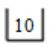→ ‘5’ is pushed in the stack→ ‘+’ comes so addition will be done by popping the top two elements in the stackand the result is pushed back into the stack, i.e., 10+5 = 15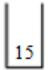→ 60 pushed in the stack→ 6 pushed in the stack→ ‘/’ comes. So, 60/6 = 10→ ‘*’ comes. So, 15 * 10 = 150→ ‘8’ comes, push in the stack→ ‘-‘ comes. So, 150-8 = 142So the final result is 142.
 Question 35

Consider a binary tree T that has 200 leaf nodes. Then, the number of nodes in T that have exactly two children are ____.

 A 199 B 198 C 197 D 196
Data-Structures       Binary-Trees       GATE 2015 [Set-3]
Question 35 Explanation:
A binary tree T with n leaf nodes have exactly (n – 1) nodes that have exactly two children.
 Question 36

Let G be a graph with n vertices and m edges. What is the tightest upper bound on the running time of Depth First Search on G, when G is represented as an adjacency matrix?

 A θ(n) B θ(n+m) C θ(n2) D θ(m2)
Data-Structures       Graphs       GATE 2014 [Set-1]
Question 36 Explanation:
DFS visits each vertex once and as it visits each vertex, we need to find all of its neighbours to figure out where to search next. Finding all its neighbours in an adjacency matrix requires O(V ) time, so overall the running time will be O(V2).
 Question 37

Consider a rooted n node binary tree represented using pointers. The best upper bound on the time required to determine the number of subtrees having exactly 4 nodes is O(nalogbn). Then the value of a+10b is ________.

 A 1 B 2 C 3 D 4
Data-Structures       Time-Complexity       GATE 2014 [Set-1]
Question 37 Explanation:
Binary tree traversal takes O(n) time for reaching 4th level from the leaf node. Every node has to check their subtree having exactly 4 nodes. Checking time can be done in maximum constant time for each node. Nodes in the level is always less than n and it’s complexity is O(n). Finally a value becomes 1 and b value becomes 0.
Substitute into a and b values in equation.
⇒ a+10b
⇒ a*1 + 10*0
⇒ 1+0
⇒ 1
 Question 38

Consider the directed graph given below.Which one of the following is TRUE?

 A The graph does not have any topological ordering. B Both PQRS and SRQP are topological. C Both PSRQ and SPRQ are topological orderings. D PSRQ is the only topological ordering.
Data-Structures       Graphs       GATE 2014 [Set-1]
Question 38 Explanation: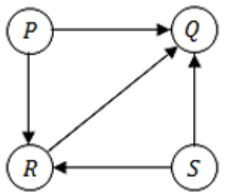There are no cycles in the graph, so topological orderings do exist.
We can consider P & S as starting vertex, followed by R & Q.
Hence, PSRQ & SPRQ are the topological orderings.
 Question 39

Let P be a quicksort program to sort numbers in ascending order using the first elements as the pivot. Let t1 and t2 be the number of comparisons made by P for the inputs [1 2 3 4 5] and [4 1 5 3 2] respectively. Which one of the following holds?

 A t1=5 B t12 C t1>t2 D t1=t2
Data-Structures       Quick-Sort       GATE 2014 [Set-1]
Question 39 Explanation:
[1, 2, 3, 4, 5] [4, 1, 5, 3, 2]
Simple one: First element is a pivot element. And if we observe first array pivot is small and requires lot of comparisons and whereas it is not the case with 2nd array through not in sorted manner.
Hence t1> t2.
 Question 40

Consider a hash table with 9 slots. The hash function is h(k) = k mod 9. The collisions are resolved by chaining. The following 9 keys are inserted in the order: 5, 28, 19, 15, 20, 33, 12, 17, 10. The maximum, minimum, and average chain lengths in the hash table, respectively, are

 A 3, 0, and 1 B 3, 3, and 3 C 4, 0, and 1 D 3, 0, and 2
Data-Structures       Hashing       GATE 2014 [Set-1]
Question 40 Explanation:
Hash table has 9 slots.
h(k) = k mod 9
Collisions are resolved using chaining.
Keys: 5, 28, 19, 15, 20, 33, 12, 17, 10.5%9 – 5
28%9 – 1
19%9 – 1
15%9 – 6
20%9 – 2
33%9 – 6
12%9 – 3
17%9 – 8
10%9 – 1
Maximum chain length is 3
Minimum chain length is 0
Average chain length = 0+3+1+1+0+1+2+0+1/ 9 = 1
 Question 41

Consider the following C function in which size is the number of elements in the array E: The value returned by the function MyX is the

```int MyX(int *E, unsigned int size)
{
int Y = 0;
int Z;
int i, j, k;
for(i = 0; i < size; i++)
Y = Y + E[i];
for(i = 0; i < size; i++)
for(j = i; j < size; j++)
{
Z = 0;
for(k = i; k <= j; k++)
Z = Z + E[k];
if (Z > Y)
Y = Z;
}
return Y;
}
```
 A maximum possible sum of elements in any sub-array of array E. B maximum element in any sub-array of array E. C sum of the maximum elements in all possible sub-arrays of array E. D the sum of all the elements in the array E.
Data-Structures       General       GATE 2014 [Set-1]
Question 41 Explanation:
Y=0
for (i=0; i Y=Y+E[i] // E is an array, this statement calculates the sum of elements of the array E and stores it in Y.
for (i=0; i for(j=i; j {
Z=0;
for(k=i; k<=j; k++)
Z=Z+E[k];
// It calculates the sum of all possible subarrays starting from 0 i.e., the loop will iterate for all the elements of array E and for every element, calculate sum of all sub arrays starting with E[i].
Store the current sum in Z.
If Z is greater than Y then update Y and return Y. So it returns the maximum possible sum of elements in any sub array of given array E.
 Question 42

Consider the following pseudo code. What is the total number of multiplications to be performed?

```D = 2
for i = 1 to n do
for j = i to n do
for k = j + 1 to n do
D = D * 3```
 A Half of the product of the 3 consecutive integers. B One-third of the product of the 3 consecutive integers. C One-sixth of the product of the 3 consecutive integers. D None of the above.
Data-Structures       Time-Complexity       GATE 2014 [Set-1]
Question 42 Explanation:
D = 2
for i = 1 to n do
for j = i to n do
for k = j + 1 to n do
D = D * 3;Also you can try for smaller ‘n’.
 Question 43

Consider a 6-stage instruction pipeline, where all stages are perfectly balanced. Assume that there is no cycle-time overhead of pipelining. When an application is executing on this 6-stage pipeline, the speedup achieved with respect to non-pipelined execution if 25% of the instructions incur 2 pipeline stall cycles is _________.

 A 4 B 5 C 6 D 7
Data-Structures       Pipelining       GATE 2014 [Set-1]
Question 43 Explanation:
For 6 stages, non- pipelining takes 6 cycles.
There were 2 stall cycles for pipelining for 25% of the instructions.
So pipeline time =(1+(25/100)*2)=3/2=1.5
Speed up =Non-pipeline time / Pipeline time=6/1.5=4
 Question 44

An access sequence of cache block addresses is of length N and contains n unique block addresses. The number of unique block addresses between two consecutive accesses to the same block address is bounded above by k. What is the miss ratio if the access sequence is passed through a cache of associativity A≥k exercising least-recently-used replacement policy?

 A n/N B 1/N C 1/A D k/n
Data-Structures       Cache       GATE 2014 [Set-1]
Question 44 Explanation:
Consider the scenario, you have k-way set associative cache, let us say a block b0 comes to set-0 and then there are k-1 unique blocks to the same set. Now the set-0 is full then one more block came to set-0 and the block b0 is replaced using LRU, if b0 comes again then it will cause a miss. But it is given that the set associativity A>=k, means the no. of blocks in a set is k or more so assume we have (k+1)-way set associativity, applying the above logic b0 comes first and then k-1 other unique accesses come to the same set-0 then there is still place for one more block in set-0 and if b0 comes again now then it will not cause a miss. As the associativity increases further there will not be any more misses other than the unique blocks we have a best case scenario. So out of N accesses only the unique blocks n can be missed and the miss ratio is n/N.
As the set associativity size keeps increasing then we don’t have to replace any block, so LRU is not of any significance here.
 Question 45

A priority queue is implemented as a Max-Heap. Initially, it has 5 elements. The level-order traversal of the heap is: 10, 8, 5, 3, 2. Two new elements 1 and 7 are inserted into the heap in that order. The level-order traversal of the heap after the insertion of the elements is:

 A 10, 8, 7, 3, 2, 1, 5 B 10, 8, 7, 2, 3, 1, 5 C 10, 8, 7, 1, 2, 3, 5 D 10, 8, 7, 5, 3, 2, 1
Data-Structures       Heap       GATE 2014 [Set-2]
Question 45 Explanation:
Max-Heap has 5 elements:The level order traversal in this max heap final is:
10, 8, 7, 3, 2, 1, 5.
 Question 46

Consider the tree arcs of a BFS traversal from a source node W in an unweighted, connected, undirected graph. The tree T formed by the tree arcs is a data structure for computing

 A the shortest path between every pair of vertices. B the shortest path from W to every vertex in the graph. C the shortest paths from W to only those nodes that are leaves of T. D the longest path in the graph.
Data-Structures       Graphs       GATE 2014 [Set-2]
Question 46 Explanation:
One of the application of BFS algorithm is to find the shortest path between nodes u and v.
But in the given question the BFS algorithm starts from the source vertex w and we can find the shortest path from W to every vertex of the graph.
 Question 47

The worst case running time to search for an element in a balanced binary search tree with n2n elements is

 A Θ (n log n) B Θ (n2n) C Θ (n) D Θ (log n)
Data-Structures       Binary-Search-Tree       GATE 2012
Question 47 Explanation:
→ Worst case running time to search for an element in a balanced binary search tree of ‘n’ elements is (log n).
→ No of elements = n.2n then search time = (log n.2n)
= (log n + log 2n)
= (log n + n log 2)
= O(n)
 Question 48

The recurrence relation capturing the optimal execution time of the Towers of Hanoi problem with n discs is

 A T(n) = 2T(n – 2) + 2 B T(n) = 2T(n – 1) + n C T(n) = 2T(n/2) + 1 D T(n) = 2T(n – 1) + 1
Data-Structures       Recursion       GATE 2012
Question 48 Explanation:
The recurrence equation for given recurrence function is
T(n) = 2T(n – 1) + 1
= 2 [2T(n – 2) + 1] + 1
= 22 T(n – 2) + 3

= 2k T( n – k) + (2k – 1)
n – k = 1
= 2n-1 T(1) + (2n-1 – 1)
= 2n-1 + 2n-1 – 1
= 2n – 1
≌ O(2n)
 Question 49

Suppose a circular queue of capacity (n – 1) elements is implemented with an array of n elements. Assume that the insertion and deletion operations are carried out using REAR and FRONT as array index variables, respectively. Initially, REAR = FRONT = 0. The conditions to detect queue full and queue empty are

 A full: (REAR+1)mod n == FRONT empty: REAR == FRONT B full: (REAR+1)mod n == FRONT empty: (FRONT+1) mod n == REAR C full: REAR == FRONT empty: (REAR+1) mod n == FRONT D full: (FRONT+1)mod n == REAR empty: REAR == FRONT
Data-Structures       Queues       GATE 2012
Question 49 Explanation: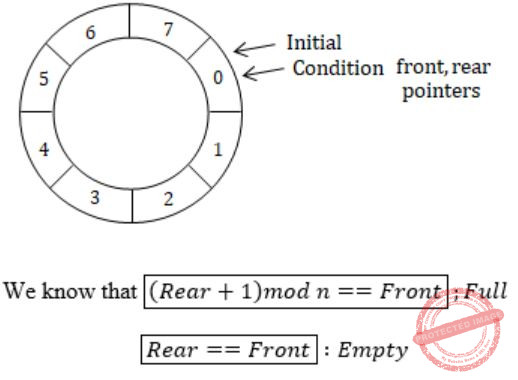To circulate within the queue we have to write mod n for (Rear + 1).
We insert elements using Rear & delete through Front.
 Question 50

The height of a tree is defined as the number of edges on the longest path in the tree. The function shown in the pseudocode below is invoked as height (root) to compute the height of a binary tree rooted at the tree pointer root.The appropriate expression for the two boxes B1 and B2 are

 A B1: (1+height(n→right)) B2: (1+max(h1,h2)) B B1: (height(n→right)) B2: (1+max(h1,h2)) C B1: height(n→right) B2: max(h1,h2) D B1: (1+ height(n→right)) B2: max(h1,h2)
Data-Structures       Binary-Trees       GATE 2012
Question 50 Explanation:Now, we analyze the code,
The box B1 gets executed when left sub tree of n is NULL & right subtree is not NULL.
In such case, height of n will be the height of the right subtree + 1.
The box B2 gets executed when both left & right subtrees of n are not NULL.
In such case, height of n will be max of heights of left & right subtrees of n+1.
There are 50 questions to complete.

Register Now